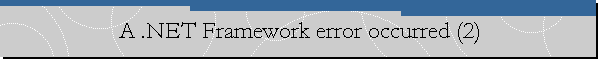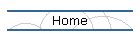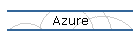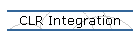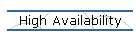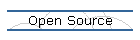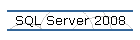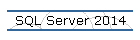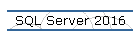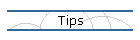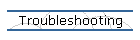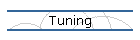## A .NET Framework error occurred during execution of user defined routine or aggregate 'geometry'.

Applies to: Microsoft SQL Server 2008 (Katmai) November CTP (CTP 5).

Error Message.

(local)(DOMAINNAME\amorillo): Msg 6522, Level 16, State 1, Line 3
A .NET Framework error occurred during execution of user defined routine or aggregate 'geometry':
System.FormatException: 24119: The Polygon input is not valid because the start and end points of the exterior ring are not the same. Each ring of a polygon must have the same start and end points.
System.FormatException:
at Microsoft.SqlServer.Types.GeometryDataBuilder.EndFigure()
at Microsoft.SqlServer.Types.SqlGeometry.STGeomFromText(SqlChars geometryTaggedText, Int32 srid)
.

(local)(DOMAINNAME\amorillo): (1 row(s) affected)Cause.

Each ring of a polygon must have the same start and end points. If you don't do this you would get the error shown above.

Solution.

Let's convert the original geometry instance into a valid one.

The original instance is the following:

Declare @shape geometry

SET @shape = geometry::STGeomFromText
('POLYGON ((0 0, 150 0, 150 150, 0 150, 150 150))',0)
SELECT @shape.ToString()

The valid instance would be:

SET @shape = geometry::STGeomFromText
('POLYGON ((0 0, 150 0, 150 150, 0 150, 0 0))',0)
SELECT @shape.ToString()